I. THE SINE WAVE AND STABILITY A sine wave signal generator produces a voltage that changes in time in a sinusoidal way as shown in figure 1.1 The signal is an oscillating signal because the sine wave repeats itself. A cycle (2 p radians of phase) of the oscillation is produced in one period "T".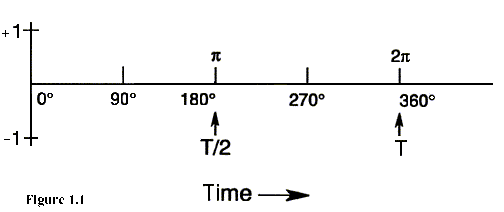Figure 1.1: A repeating sine function is the basis of an oscillating signal. It is convenient for us to express angles in radians rather than in units of degrees, and positive zero-crossings of the voltage will occur every 2 p radians. The frequency "n " is the number of cycles in one second (Hz), which is the reciprocal of period (seconds per cycle). The expression describing the voltage "V" out of a sine wave signal generator is given by V(t) = V0[1 + a(t)]sin[F (t)] where V0 is the peak voltage amplitude, and F (t) is the total accumulated phase. Equivalent expressions are: V(t)=V0[1+a(t)]sin(2pt / T) and V(t)=V0[1+a(t)]sin(2pvt) For the following discussion, we will assume the amplitude noise a(t) is zero. Consider figure 1.2. Let's assume that the maximum value of "V" equals 1, hence "V0" = 1. We say that the voltage "V(t)" is normalized to unity.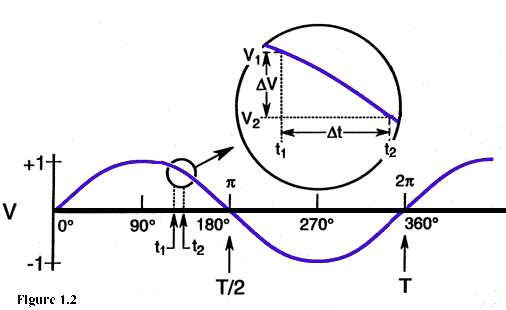Figure 1.2: For a given phase, DV vs. Dt of the sine-wave signal corresponds to a unique minimum frequency called the instantaneous frequency if Dt is diminishingly small. . If we are given the frequency of the sinewave, then no matter how big or small D t may be, we can determine D V. Let us look at this from another point of view. Suppose we can measure D V and D t. From this, there is a sine wave at a unique minimum frequency corresponding to the given D V and D t. For infinitesimally small D t, this frequency is called the instantaneous frequency at this t. The smaller the interval D t, the better the approximation of instantaneous frequency at t. In practice, because of finite bandwidths, we cannot measure the instantaneous frequency.  When we speak of oscillators and the signals they produce, we recognize that an oscillator has some nominal frequency at which it operates. The "frequency stability" of an oscillator is a term used to characterize how small the frequency fluctuations are of the oscillator signal. The IEEE now has a formal definition for "frequency stability". One usually refers to frequency stability when comparing one oscillator with another. As we shall see later, we can define particular aspects of an oscillator's output then draw conclusions about its relative frequency stability. People often speak of "frequency stability" when they mean "frequency instability." Frequency stability is the degree to which an oscillating signal produces the same value of frequency for any interval, D t, throughout a specified period of time. An internationally recommended definition of "frequency instability" is: "The spontaneous and/or environmentally caused frequency change within a given time interval." Let's examine the two waveforms shown in figure 1.3. Frequency stability depends on the amount of time involved in a measurement. Of the two oscillating signals, it is evident that "2" is more stable than "1" from time t1 to t3 assuming the horizontal scales are linear in time. From time t1 to t2, there may be some question as to which of the two signals is more stable, but it's clear that from time t2 to t3, signal "1" is at a different frequency from that in interval t1 to t2.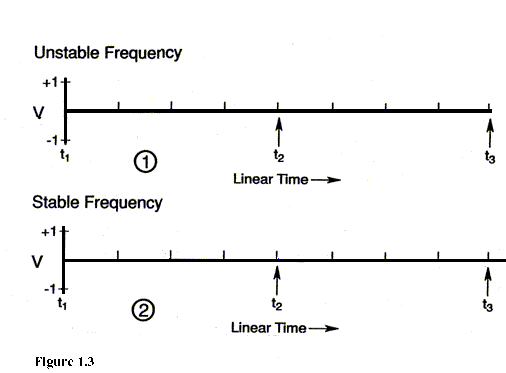Figure 1.3: Top: Instantaneous frequency is inconsistent and less stable from t2 to t3. Bottom: Instantaneous frequency is consistent and more stable throughout. If we want an oscillator to produce a particular frequency n 0, then we're correct in stating that if the oscillator signal frequency deviates from n 0 over any interval, this is a result of something which is undesirable. In the design of an oscillator, it is important to consider the sources of mechanisms which degrade the oscillator's frequency stability. These undesirable mechanisms cause random (noise) or systematic processes to exist on top of the sine wave signal of the oscillator. To account for the noise components at the output of a sine wave signal generator, we can express the output as V(t)=V0[1+a(t)]sin(2v0t+f(t)) (1.1) where V0 = nominal peak voltage amplitude, a(t) = deviation of amplitude from nominal, i.e., d V/V0 n 0 = nominal fundamental frequency, f (t) = deviation of phase from nominal. Ideally "a" and " f " should equal zero for all time. However, in the real world there are no perfect oscillators. To determine the extent of the noise components "a" and "f ", we shall turn our attention to measurement techniques. The typical precision oscillator, of course, presumably has a stable sinusoidal voltage output with a frequency n and a period of oscillation T, which is the reciprocal of the frequency (n = 1/T). One goal is to measure the frequency and/or the frequency stability of the sinusoid. Instability is actually measured, but with little confusion it is often called stability in the literature. Naturally, fluctuations in frequency correspond to fluctuations in the period. Almost all frequency measurements, with very few exceptions, are measurements of phase or of the period fluctuations in an oscillator, not of frequency, even though the frequency may be the readout. As an example, most frequency counters sense the zero (or near zero) crossing of the sinusoidal voltage, which is the point at which the voltage is the most sensitive to phase fluctuations. One must also realize that any frequency measurement involves two oscillators. In some instances, one oscillator is in the counter. It is impossible to purely measure only one oscillator. In some instances one oscillator may be enough better than the other that the fluctuations measured may be considered essentially those of the latter. However, in general because frequency measurements are always dual, it is useful to define: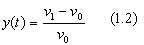as the fractional frequency difference or deviation of oscillator one, n 1, with respect to a reference oscillator n 0 divided by the nominal frequency n 0. Conceptually, we can also think of eq. (1.2) as the free running frequency of an individual oscillator, n 1, differenced with its own nominal value, n 0. Now, y(t) is a dimensionless quantity and useful in describing oscillator and clock performance; eg., the time deviation, x(t), of an oscillator over a period of time, t, is simply given by: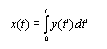(1.3) We see that the time deviations and the phase deviations are related by a constant, 1/2 p n 0. Since it is impossible to measure instantaneous frequency, any frequency or fractional frequency measurement always involves some sample time, D t or "t "--some time window through which the oscillators are observed; whether it's a picosecond, a second, or a day, there is always some sample time. So when determining a fractional frequency, y(t), in fact what is happening is that the time deviation is being measured say starting at some time t and again at a later time, t + t . The difference in these two time deviations divided by t gives the average fractional frequency over that period t :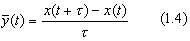Tau, t , may be called the sample time or averaging time; it may be determined, for example, by the gate time of a counter. What happens in many cases is that one samples a number of cycles of an oscillation during the preset gate time of a counter; after the gate time has elapsed, the counter latches the value of the number of cycles so that it can be read out, printed, or stored in some other way. Then there is a delay time for such processing of the data before the counter arms and starts again on the next cycle of the oscillation. During the delay time (or process time), information is lost. We have chosen to call it dead time and in some instances it becomes a problem. Unfortunately for data processing in typical oscillators the effects of dead time often hurt most when it is the hardest to avoid. In other words, for times that are short compared to a second when it is very difficult to avoid dead time, that is usually where dead time can make a significant difference in the data analysis. Typically, for many oscillators, if the sample time is long compared to a second, the dead time makes little difference in the data analysis, unless it is excessive.1 New equipment or techniques are now available which contribute zero or negligible dead time.2 In reality, of course, the sinusoidal output of an oscillator is not pure, but it contains noise (frequency) fluctuations as well. This section deals with the measurement of these fluctuations to determine the quality of a precision signal source. We will describe five different methods of measuring the frequency fluctuations in precision oscillators which do not include measuring the frequency directly in a frequency counter. The direct frequency counter technique is often very limiting because the number of resolvable digits on the counter are often inadequate for precision oscillators.   1.1 Common Methods of Measuring Frequency Stability A. Beat frequency method The first technique is called a heterodyne frequency measuring method or beat frequency method. The signal from two independent oscillators are fed into the two ports of a double balanced mixer as illustrated in figure 1.4.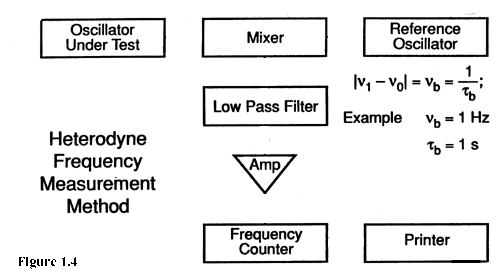Figure 1.4: Measurement of the frequency difference (�beat note�) between oscillators can increase measurement precision. State-of-the-art oscillators can readily be measured by this method. The difference frequency or the beat frequency, n b, is obtained as the output of a low pass filter which follows the mixer. This beat frequency is then amplified and fed to a frequency counter and printer or to some recording device. The fractional frequency is obtained by dividing n b by the nominal carrier frequency n 0. This system has excellent precision; one can measure essentially all state-of-the-art oscillators. B. Dual mixer time difference (DMTD) system This system has proved to be very popular. A block diagram is shown in figure 1.5. To preface the remarks on the DMTD, it should be mentioned that if the time or the time fluctuations can be measured directly, an advantage is obtained over just measuring the frequency. The reason is that one can calculate the frequency from the time without dead time as well as know the time behavior. The reason, in the past, that frequency has not been inferred from the time (for sample times of the order of several seconds and shorter) is that the time difference between a pair of oscillators operating as clocks could not be measured with sufficient precision. The system described in this section has demonstrated a precision of 10-13 seconds. Such precision opens the door to making time measurements as well as frequency and frequency stability measurements for sample times as short as a few milliseconds and longer, all without dead time.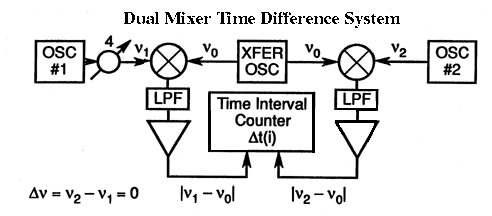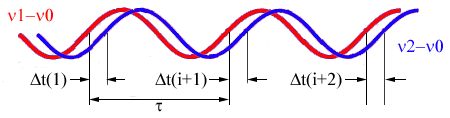Figure 1.5: Measurement of the time difference between two beat notes from two oscillators with a common transfer oscillation can further increase measurement precision. Instability of transfer oscillator cancels to first order. In figure 1.5, oscillator 1 could be considered under test and oscillator 2 could be considered the reference oscillator. These signals go to the ports of a pair of double balanced mixers. Another oscillator with separate symmetric buffered outputs is fed to the remaining other two ports of the pair of double balanced mixers. This common oscillator's frequency is offset by a desired amount from the other two oscillators. Then two different beat frequencies come out of the two mixers as shown. These two beat frequencies will be out of phase by an amount proportional to the time difference between oscillator 1 and 2--excluding the differential phase shift that may be inserted. Further, the beat frequencies differ in frequency by an amount equal to the frequency difference between oscillators 1 and 2. This measurement technique is very useful where oscillator 1 and oscillator 2 are very near the same frequency. This is typical for atomic standards (cesium, rubidium, and hydrogen frequency standards). Illustrated at the bottom of figure 1.5 is what might represent the beat frequencies out of the two mixers. A phase shifter may be inserted as illustrated to adjust the phase so that the two beat rates are nominally in phase; this adjustment sets up the nice condition that the noise of the common oscillator tends to cancel (for certain types of noise) when the time difference is determined. After amplifying these beat signals, the start port of a time interval counter is triggered with the positive zero crossing of the other beat. Taking the time difference between the zero crossings of these beat frequencies, one measures the time difference between oscillator 1 and oscillator 2, but with a precision which has been amplified by the ratio of the carrier frequency to the beat frequency (over that normally achievable with this same time interval counter). The time difference x(i) for the ith measurement between oscillators 1 and 2 is given by eq (1.5).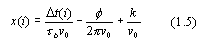where D t(i) is the ith time difference as read on the counter, t b is the beat period, n 0 is the nominal carrier frequency, f is the phase delay in radians added to the signal of oscillator 1, and k is an integer to be determined in order to remove the cycle ambiguity. It is only important to know k if the absolute time difference is desired; for frequency and frequency stability measurements and for time fluctuation measurements, k may be assumed zero unless one goes through a cycle during a set of measurements. The fractional frequency can be derived in the normal way from the time fluctuations.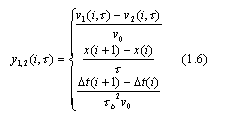In eqs (1.5) and (1.6), assumptions are made that the transfer (or common) oscillator is set at a lower frequency than oscillators 1 and 2, and that the voltage zero crossing of the beat n 1 - n c starts and that n 2 - n c stops the time interval counter. The fractional frequency difference may be averaged over any integer multiple of t b:where m is any positive integer. If needed, t b can be made to be very small by having very high beat frequencies. The transfer (or common) oscillator may be replaced with a low phase-noise frequency synthesizer, which derives its basic reference frequency from oscillator 2. In this set-up the nominal beat frequencies are simply given by the amount that the output frequency of the synthesizer is offset from n 2. Sample times as short as a few milliseconds are easily obtained. Logging the data at such a rate can be a problem without special equipment. The latest NBS (now NIST) time scale measurement system is based on the DMTD and is yielding an excellent cost benefit ratio. C. Loose phase lock loop method This first type of phase lock loop method is illustrated in figure 1.6. The signal from an oscillator under test is fed into one port of a mixer. The signal from a reference oscillator is fed into the other port of this mixer. The signals are in quadrature, that is, they are 90 degrees out of phase so that the average voltage out of the new mixer is nominally zero, and the instantaneous voltage fluctuations correspond to phase fluctuations rather than to amplitude fluctuations between the two signals. The mixer is a key element in the system. The advent of the Schottky barrier diode was a significant breakthrough in making low noise precision stability measurements. The output of this mixer is fed through a low pass filter and then amplified in a feedback loop, causing the voltage controlled oscillator (reference) to be phase locked to the test oscillator. The response time of the loop is adjusted such that a very loose phase lock (long time constant) condition exists. This is discussed later in section VIII.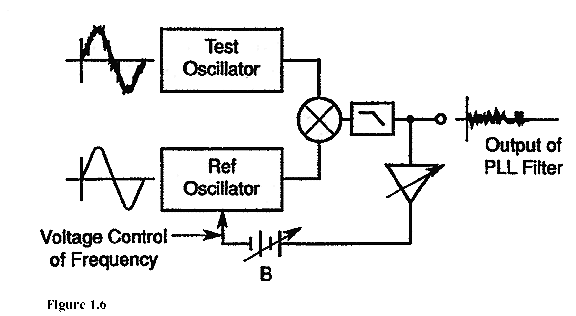Figure 1.6: Direct measurement of the phase difference between two oscillators yields excellent precision. The technique requires electronic frequency control of a clean reference oscillator to maintain a loose phase lock, hence a zero beat. The response (or attack) time is the time it takes the servo system to make 70% of its ultimate correction after being slightly disturbed. The response time is equal to 1/ p wh, where wh is the servo bandwidth. If the response time of the loop is about a second, then the voltage fluctuations will be proportional to the phase fluctuations for sample time shorter than one second. Depending on the coefficient of the tuning capacitor and the quality of the oscillators involved, the amplification used may vary significantly but may typically range from 40 to 80 dB via a good low noise amplifier. In turn this signal can be fed to a spectrum analyzer to measure the Fourier components of the phase fluctuations. This system of frequency-domain analysis is discussed in sections VIII to X. It is of particular use for sample times shorter than one second (for Fourier frequencies greater than 1 Hz) in analyzing the characteristics of an oscillator. It is specifically very useful if one has discrete side bands such as 60 Hz or detailed structure in the spectrum. How to characterize precision oscillators using this technique will be treated in detail later in section IX and XI. One may also take the output voltage from the above amplifier and feed it to an A/D converter. This digital output becomes an extremely sensitive measure of the short term time or phase fluctuations between the two oscillators. Precisions of the order of a picosecond are easily achievable. D. Tight phase lock loop method The second type of phase lock loop method (shown in figure 1.7) is essentially the same as the first in figure 1.6 except that in this case the loop is in a tight phase lock condition; i.e., the response time of the loop is much shorter than the sample times of interest--typically a few milliseconds. In such a case, the phase fluctuations are being integrated so that the voltage output is proportional to the frequency fluctuations between the two oscillators and is no longer proportional to the phase fluctuations (for sample times longer than the response time of the loop). A bias box is used to adjust the voltage on the varicap to a tuning point that is fairly linear and of a reasonable value. The voltage fluctuations prior to the bias box (biased slightly away from zero) may be fed to a voltage to frequency converter which in turn is fed to a frequency counter where one may read out the frequency fluctuations with great amplification of the instabilities between this pair of oscillators. The frequency counter data are logged with a data logging device. The coefficient of the varicap and the coefficient of the voltage to frequency converter are used to determine the fractional frequency fluctuations, yi, between the oscillators, where i denotes the ith measurement as shown in figure 1.7. It is not difficult to achieve a sensitivity of a part in 1014 per Hz resolution of the frequency counter, so one has excellent precision capabilities with this system.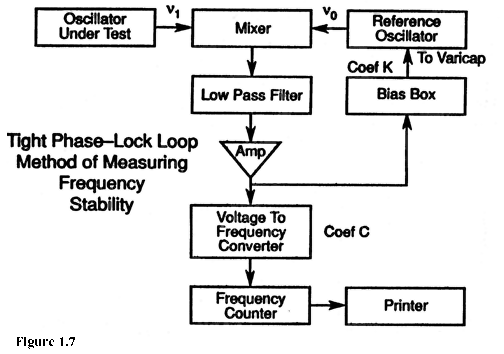E. Time difference method The last measurement method we will illustrate is very commonly used, but typically does not have the measurement precision more readily available in the first four methods illustrated above. This method is called the time difference method and is shown in figure 1.8. Because of the wide bandwidth needed to measure fast rise-time pulses, this method is limited in signal-to-noise ratio. However, some counters are commercially available allowing one to do signal averaging or to do precision rise-time comparison (precision of time difference measurements in the range of 10 ns to 10 ps are now available). Such a method yields a direct measurement of x(t) without any translation, conversion, or multiplication factors. Caution should be exercised in using this technique even if adequate measurement precision is available because it is not uncommon to have significant instabilities in the frequency dividers shown in figure 1.8--of the order of a few nanoseconds. The technology exists to build better frequency dividers than are commonly available, but manufacturers have not yet availed themselves of state-of-the-art techniques in a cost beneficial manner. A trick to bypass divider problems is to feed the oscillator signals directly into the time interval counter and observe the zero voltage crossing into a well matched impedance. (In fact, in all of the above methods one needs to pay attention to impedance matching, cable lengths and types, and connectors). The divided signal can be used to resolve cycle ambiguity of the carrier, otherwise the carrier phase at zero volts may be used as the time reference. The slope of the signal at zero volts is 2 p V0/t 1, where t 1 = 1/n 1 (period of oscillation). For V0 = 1 volt and a 5 MHz signal, this slope is 3m volts/ns, which is a very good sensitivity.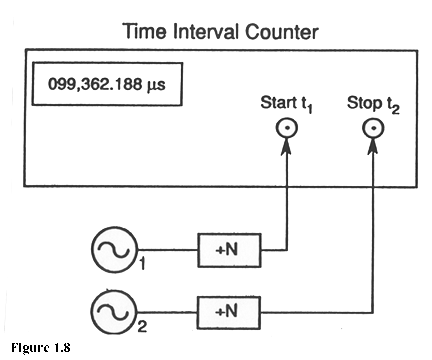Figure 1.8: Measurement of the time difference between two oscillators, usually after division by N to obtain 1 pulse-per-second, yields only moderate measurement performance compared to previous methods. The technique is dependent on several properties of the counter and its trigger circuits. Main Page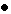Table of ContentsGo to section: Summary and IntroductionIIIIIIIVVVIVIIVIIIIXXXIXIIConclusionReferences